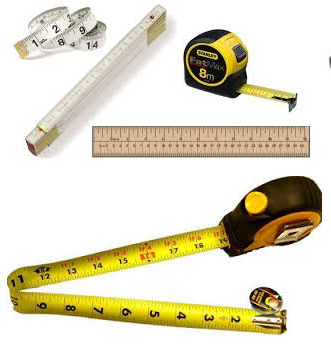# Unit Conversion

##Civil Unit Conversion Calculator

meter

#### Unit Conversion

1.00 Metre is same as

Sr. Unit Value
1 Feet 3.28
2 Kilometre 0.00
3 Mile 0.00
4 Yard 1.09
5 Inch 39.37
6 Centimetre 100.00

## What is Unit Conversion?

Unit conversion is a multi-step process that involves multiplication or division by a numerical factor, selection of the correct number of significant digits, and rounding.

Length Conversion describe between the various different sizes by merely moving the decimal point the correct number of places. The basic metric units are meters (for length).

Area Conversion describes the total amount of space a 2 dimensional shape covers. The units used to describe an area are based on the length of a side of a square, the standard SI unit for area is the square meter.

Volume conversion a space any substance occupies or contains in a three dimensional space. While the Si unit for volume is the cubic meter, it is too big for common use and has been replaced with the liter (1 cubic decimeter) in everyday life.## Length Conversion Table

Unit Name Converted Value
1 metre (m) 3.2808 feet (ft)
1 kilometre (km) 1000 metre (m)
1 mile 1.609 kilometre (km)
1 feet (ft) 12 inch
1 inch 2.54 centimetre (cm)
1 yard 3 feet (ft)

## Volume Conversion Table

Unit Name Converted Value
1 Cubic metre (m3) 35.3147 Square feet (ft3)
1 Cubic metre (m3) 61023.7 Cubic Inch
1 Cubic Feet (ft3) 1728 Cubic Inch

## Area Conversion Table

Unit Name Converted Value
1 Square metre (m2) 10.7639 Square feet (ft2)
1 Square metre (m2) 1.1959 Square yard
1 Square Yard 9 Square feet (ft2)
1 Hectare 2.4711 Acre
1 Hectare 10000 Square metre (m2)
1 Hectare 107639 Square feet (ft2)
1 Acre 4046.86 Square metre (m2)
1 Guntha 121 Square Yard
1 Vigha 16 Guntha
1 Vigha 1618.73 Square metre (m2)
1 Vigha 17423.9124 Square feet (ft2)
1 Acre 40 Guntha
1 Hectare 10000 Square metre (m2)